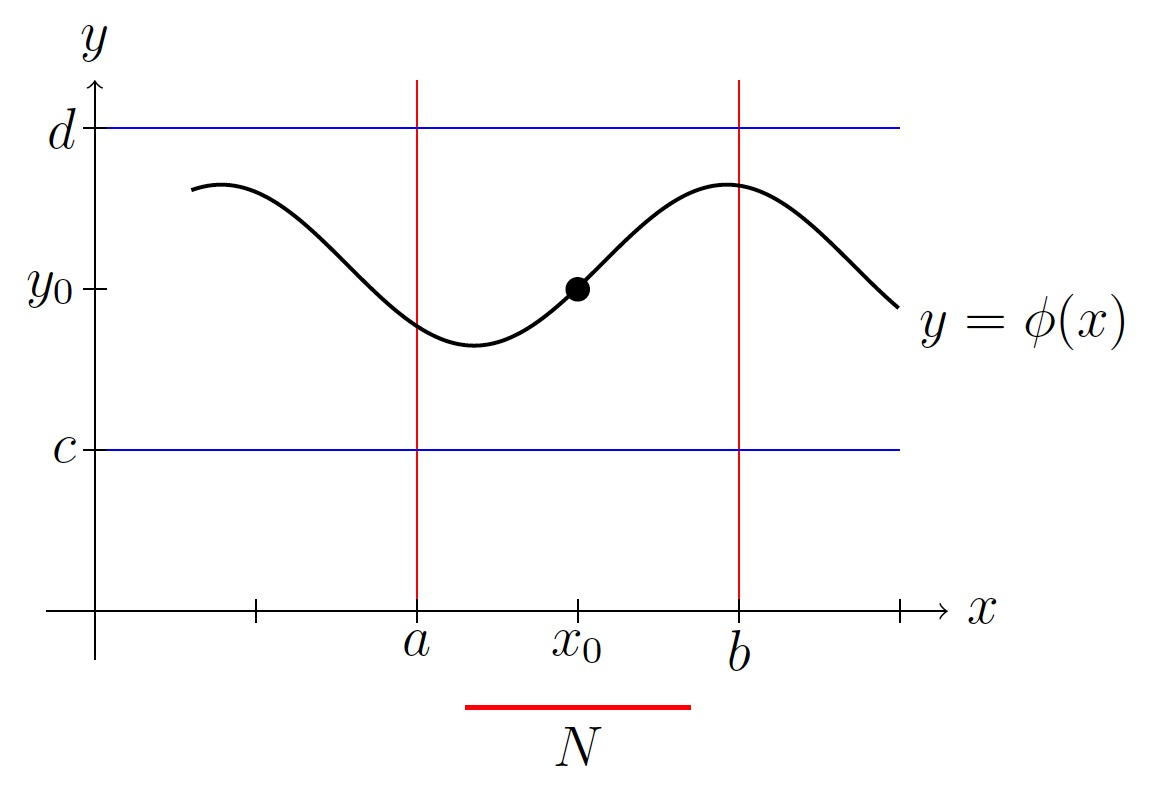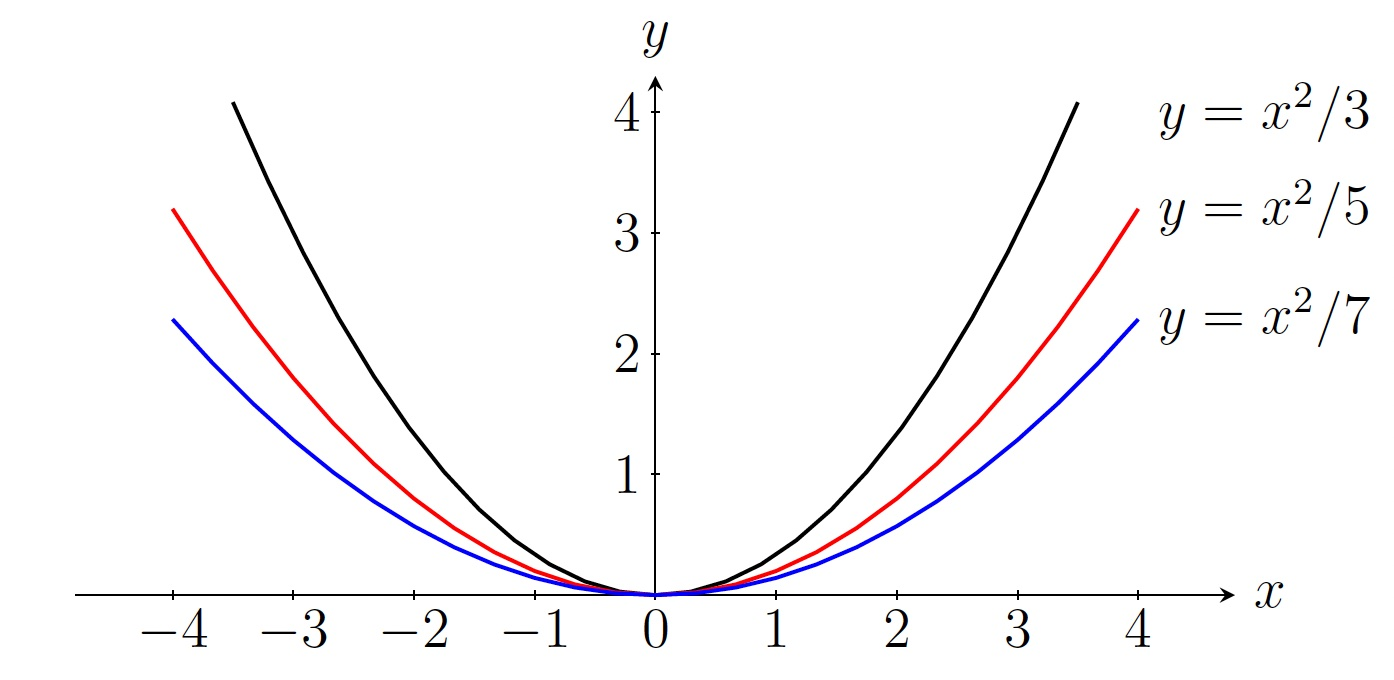DiffEq Home

## Existence and Uniqueness of Solutions

We ask two questions for the following ODE $\displaystyle\frac{dy}{dx}=f(x,y),\, y(x_0)=y_0 \;\;\;\;(21)$

1. Is there any solution to (21)? (existence of solution?)

2. Is there a unique solution to (21)? (uniqueness of solution?)

The answers are given by the Existence and Uniqueness Theorem:
Theorem.

1. If $$f$$ is a continuous function on a neighborhood $$(a,b)\times (c,d)$$ of $$(x_0,y_0)$$, then there is a solution $$y=\phi(x)$$ to (21) in a neighborhood $$N$$ of $$x_0$$ where $$N$$ is inside $$(a,b)$$.

2. If $$f$$ and $$\displaystyle\frac{\partial f}{\partial y}$$ are continuous functions on a neighborhood $$(a,b)\times (c,d)$$ of $$(x_0,y_0)$$, then there is a unique solution $$y=\phi(x)$$ to (21) in a neighborhood $$N$$ of $$x_0$$ where $$N$$ is inside $$(a,b)$$.Example. $y'=\frac{2y}{x},\, y(1)=3$

1. Determine if the above ODE has a solution.

2. Find the largest interval in which the following ODE has a unique solution.

Solution. (a) Since $$f(x,y)=\displaystyle\frac{2y}{x}$$ is continuous function for all values of $$(x,y)$$ where $$x\neq 0$$, the give ODE has a solution $$y=\phi(x)$$ in any neighborhood of $$1$$ not containing $$0$$.

(b) $$f(x,y)=\displaystyle\frac{2y}{x}$$ and $$\displaystyle\frac{\partial f}{\partial y}=\displaystyle\frac{2}{x}$$ are continuous functions for all values of $$(x,y)$$ where $$x\neq 0$$. Because of the initial condition $$y(1)=3$$, the given ODE has a unique solution $$y=\phi(x)$$ in the interval $$(0,\infty)$$ (which is the largest possible neighborhood of $$1$$ for $$y=\phi(x)$$).

Remark.

1. If the initial condition were $$y(-1)=3$$, then the largest interval would be $$(-\infty,0)$$ for the unique solution $$y=\phi(x)$$.

2. Solving the ODE $$y'=\frac{2y}{x}$$, we get $$y=cx^2$$. So $$y=cx^2$$ is a solution of $$y'=\frac{2y}{x}$$ for each value of $$c$$.

1. If the initial condition were $$y(0)=3$$, then there is no solution which makes sense because $$f(x,y)=\displaystyle\frac{2y}{x}$$ is not continuous at $$(0,3)$$.

2. If the initial condition were $$y(0)=0$$, then there is infinitely many solutions of the form $$y=cx^2$$. It is justified because $$f(x,y)=\displaystyle\frac{2y}{x}$$ and $$\displaystyle\frac{\partial f}{\partial y}=\displaystyle\frac{2}{x}$$ are not continuous at $$(0,0)$$.

3. If the initial condition were $$y(x_0)=y_0$$ where $$x_0\neq 0$$, then there is a unique solution $$y=\frac{y_0}{x_0^2}x^2$$. It is justified because $$f(x,y)=\displaystyle\frac{2y}{x}$$ and $$\displaystyle\frac{\partial f}{\partial y}=\displaystyle\frac{2}{x}$$ are continuous at and near $$(x_0,y_0)$$ where $$x_0\neq 0$$.Example. Find the largest interval in which the following ODE has a unique solution. Justify your answer. $(t-1)(t-3)y'-2y=0,\, y(2)=-5$ Solution. $y'=\frac{2y}{(t-1)(t-3)},\, y(2)=-5$ $$f(t,y)=\displaystyle\frac{2y}{(t-1)(t-3)}$$ and $$\displaystyle\frac{\partial f}{\partial y}=\displaystyle\frac{2}{(t-1)(t-3)}$$ are continuous functions for all values of $$(t,y)$$ where $$t\neq 1,3$$. Because of the initial condition $$y(2)=-5$$, the given ODE has a unique solution $$y=\phi(t)$$ in the interval $$(1,3)$$ (which is the largest possible neighborhood of $$2$$ for $$y=\phi(t)$$).

Last edited